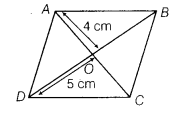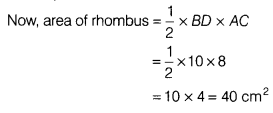# In the given figure, find the area of rhombus ABCD, if AO = 4 cm and OD = 5 cm

In the given figure, find the area of rhombus ABCD, if AO = 4 cm and OD = 5 cm.Given, ABCD is a rhombus, AO = 4 cm and OD = 5 cm.
Clearly, BD = 2OD
[∵ diagonals of rhombus bisect each other]
∴ BD =2 x 5= 10 cm
Similarly, AC = 8 cm